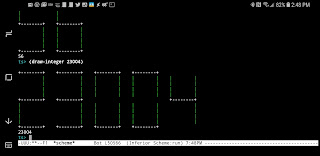## Monday, April 23, 2018

### A Little Scheme Setup and Development on the Galaxy S9 Plus

Today's goal was to setup a Scheme programming environment on my new Galaxy S9 Plus phone. I planned to use the same tools I'd used on my LG G6:

• Termux - provides a Unixy environment, doesn't require root and is just plain awesome
• tinyscheme - provides a lightweight, yet powerful, scheme environment. For bonus points, it's trivial to install within Termux. Just type: pkg install tinyscheme
• emacs, vim, git - these tools run well under Termux and are installed using the pkg install command. I use the same emacs config on my phone as I do my desktop.
• External Keyboard Helper - remaps keys on my hardware keyboard. Specifically, I use it to map Caps Lock to Escape. I've found that this keybinding is essential to making bash, emacs and vim work in a sane way.

With the tools installed, I was able to open up a new .scm file in emacs, type C-u M-x run-scheme tinyscheme and I was off and running with an interactive Scheme development environment.

While the tools installed without issue, I couldn't consider the environment setup until I wrote some code. So off I went to ProgrammingPraxis.com to find an interesting exercise to tackle.

Sticking with the theme of rendering text in unique ways, I decided to complete the Seven-Segment Devices exercise. A Seven-Segment Device is a primitive display that renders numbers and text using seven different lit up bars. Think old school calculators. Here are the seven different segments you have to work with:

``` --2--
|     |
3     4
|     |
--1--
|     |
5     6
|     |
--0--
```

Below you'll find my solution that turns any integer into text that's rendered using this seven-segment style. The exercise called for storing the data as a bit-array. I took an alternative approach, and mapped every digit in the number to a list of enabled segments. For example, 0 maps to (2 4 6 0 5 3). This is more verbose than packing the data into bits, but I was more interested in rendering the text than storing a compact representation of it.

Here are some screenshots of the code running, and the code itself is below. It felt good to be coding on my new phone, and it sure is remarkable how well all these tools Just Work.```;; https://programmingpraxis.com/2018/02/27/seven-segment-devices/

(define (show . args)
(display args)
(newline))

(define (range lower upper)
(if (< lower upper)
(cons lower (range (+ 1 lower) upper))
'()))

(define *digit-width* 10)
(define *digit-height* 11)
(define *digit-sep* 4)

(define *digit-map*
'((0 . (2 4 6 0 5 3))
(1 . (4 6))
(2 . (2 4 1 5 0))
(3 . (2 4 1 6 0))
(4 . (3 1 4 6))
(5 . (2 3 1 6 0))
(6 . (3 5 0 6 1))
(7 . (2 4 6))
(8 . (0 1 2 3 4 5 6))
(9 . (2 4 1 3 6))))

(define (integer->bars val)
(if (= val 0)
(cdr (assoc 0 *digit-map*))
(let loop ((val val) (bars '()))
(cond ((<= val 0)
bars)
(else
(let ((d (modulo val 10)))
(loop
(inexact->exact (floor (/ val 10)))
(cons (cdr (assoc d *digit-map*)) bars))))))))

(define (draw-sep)
(for-each (lambda (i)
(display " "))
(range 0 *digit-sep*)))

(define (draw first mid last)
(display first)
(for-each (lambda (i)
(display mid))
(range 0 (- *digit-width* 2)))
(display last))

(define (draw-row digits row)
(define mid (/ (- *digit-height* 1) 2))
(define top? (= row 0))
(define bottom? (= row (- *digit-height* 1)))
(define mid? (= row mid))
(define upper? (and (not top?) (< row mid)))
(define lower? (and (not bottom?) (> row mid)))
(for-each (lambda (bars)
(define (has? x) (member x bars))
(cond ((and top? (has? 2))
(draw "+" "-" "+"))
((and bottom? (has? 0))
(draw "+" "-" "+"))
((and mid? (has? 1))
(draw "+" "-" "+"))
((and upper? (has? 3) (has? 4))
(draw "|" " " "|"))
((and upper? (has? 3))
(draw "|" " " " "))
((and upper? (has? 4))
(draw " " " " "|"))
((and lower? (has? 5) (has? 6))
(draw "|"   " "   "|"))
((and lower? (has? 5))
(draw "|" " " " "))
((and lower? (has? 6))
(draw " " " " "|"))
(else
(draw " " " " " ")))
(draw-sep))
digits)
(newline))

(define (draw-integer x)
(define s (integer->bars x))
(newline)
(for-each (lambda (row)
(draw-row s row))
(range 0 *digit-height*))
x)
```